Courses

# Test: Henry's Law

## 29 Questions MCQ Test Chemistry Class 12 | Test: Henry's Law

Description
This mock test of Test: Henry's Law for Class 12 helps you for every Class 12 entrance exam. This contains 29 Multiple Choice Questions for Class 12 Test: Henry's Law (mcq) to study with solutions a complete question bank. The solved questions answers in this Test: Henry's Law quiz give you a good mix of easy questions and tough questions. Class 12 students definitely take this Test: Henry's Law exercise for a better result in the exam. You can find other Test: Henry's Law extra questions, long questions & short questions for Class 12 on EduRev as well by searching above.
QUESTION: 1

### Only One Option Correct Type This section contains 11 multiple choice questions. Each question has four choices (a), (b), (c) and (d), out of which ONLY ONE is correct An unopened soda has an aqueous concentration of C02 at 25° C equal to 0.0506 molal. Thus, pressure of C02 gas in the can is (KH = 0.034 mol/kg bar)

Solution: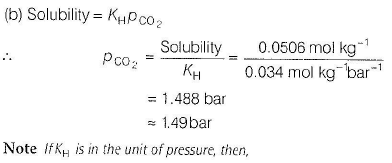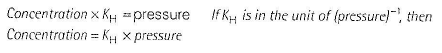QUESTION: 2

### CO(g)is dissolved in H2Oat 25°C and 0.010 atm. Henry’s law constant for this system is 5.80 x104 atm. Thus, mole fraction of CO(g)is

Solution:

The mole fraction of a gas in solution is related to partial pressure of the gas above the solution by Henry’s law.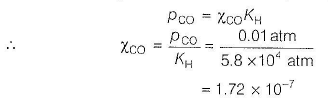QUESTION: 3

### H2S gas is used in qualitative analysis of inorganic cations. Its solubility in water at STP is 0.195 mol kg-1. Thus, Henry’s law constant (in atm molar-1) for H2S is

Solution: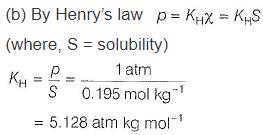QUESTION: 4

Variation of Kh (Henry’s law constant) with temperature T is shown by following graphs I - IV.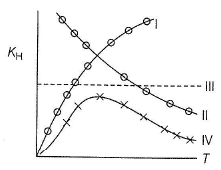Correct representation is

Solution:

Partial pressure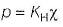If temperature is lowered, solubility of gases increases at a given pressure. Thus, KH increases with increase in temperature. It is due to this reason that aquatic species are more comfortable in cold water rather than in warm water.

QUESTION: 5

Concentration of C02 (in mole fraction) in fat when partial pressure of C02 is 55 kPa at 25° C, is (Henry’s law constant of C02 = 8.6 x 104 torr)

Solution:

Note: Kh (Henry’s law constant) is in pressure unit, hence we use relation, Concentration xKH = Pressure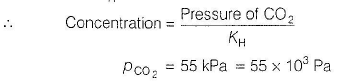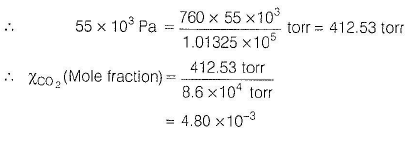QUESTION: 6

What is the concentration of 02 in a fresh water stream in equilibrium with air at 25° C and 1.0 bar? Given, KH (Henry’s law constant) of 02 = 1.3 x 10-3 mol/kg bar at 25° C.

Solution:

In this case, KH has been given in the unit of mol/kg bar. Thus, solubility of 02 (or concentration) = KH Pgas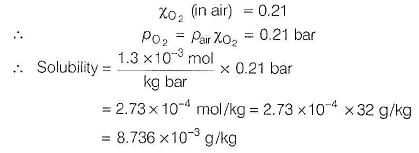QUESTION: 7

Following data has been given for C02 for the concentration in H20.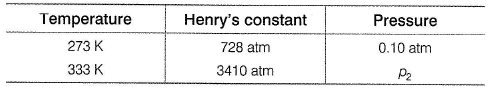If solution of C02 in H20 is heated from 273 to 333 K, pressure ( p2 ) needed to keep C02 in the solution is

Solution: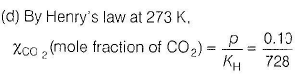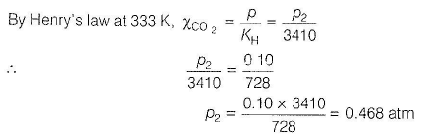QUESTION: 8

Relation between the volume of gas (2) that dissolves in a fixed volume of solvent(1) and the partial pressure of gas (2) is (nt = total moles, K1and K2 are Henry’s constants)

Solution: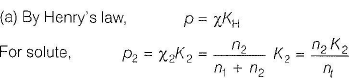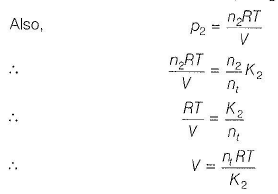QUESTION: 9

Which is correct about Henry's law

Solution:
QUESTION: 10

Henry’s law constant for N2 at 293 K is 76.48 kbar. N2 exerts a partial pressure of 0.987 bar. If N2 gas is bubbled through water at 293 K, then number of millimoles of N2 that will dissolve in 1 L of water is

Solution:

Henry’s law constant is in the unit of pressure, hence we use relation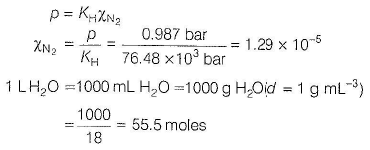Let number of moles of nitrogen = n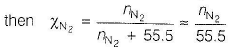(Since,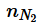is very small)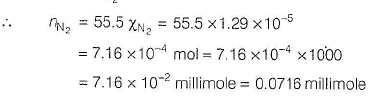QUESTION: 11

A handbook lists the solubility of carbon monoxide in water at 0° C and 1 atm pressure as 0.0354 mL CO per mL of H20. What should be the pressure of CO(g) above the solution to obtain 0.010 M CO solution?

Solution:

Volume of CO = 0 .0354 mL = 0 .0354 x 10- 3 L

p = 1 atm, T = 273 K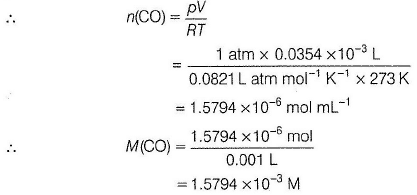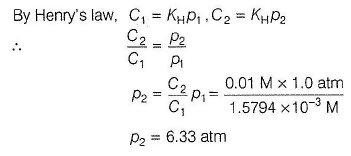*Multiple options can be correct
QUESTION: 12

One or More than One Options Correct Type
This section contains 2 multiple type questions. Each question has 4 choices (a), (b), (c) and (d), out of which ONE or MORE THAN ONE are correct
.

Select the correct statement(s).

Solution: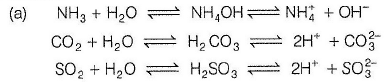NH3, C02, S03 ...... gases form ions in water, hence these gases are soluble in water. 02, N2 .......do not form ions in water hence, insoluble thus correct.

(b) NH3 and C02 are liquefied easily hence, soluble in water thus, correct.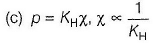Higher the value of KH, smaller the solubility (x) of the gas at a given pressure thus, correct.

*Multiple options can be correct
QUESTION: 13

Select the correct statement(s).

Solution:

(a) At constant temperature, different gases have different KH values. Thus, KH is a function of nature of gases.
Thus, correct.
(b) p (partial pressure)  =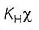If p is constant and KH value increases then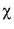(solubility) decreases. Thus, correct.
(c) KH increases with increase in temperature thus, correct.
(d) KH is independent of pressure thus, incorrect.

*Multiple options can be correct
QUESTION: 14

Statement Type
This section is based on Statement I and Statement II. Select the correct anser from the codes given below
Statement I Solubility of gases in liquids decreases with rise in temperature.
Statement II CO2(g) + H20 ( / ) ----- » H2CO3(aq ); ΔH = - ve

Solution:

(b) Dissolution of gas can be considered as the reverse of vaporisation, i.e. condensation in which heat is evolved. It is thus, exothermic ΔH < 0. By Le-Chatelier’s principle, increase in temperature shifts the dissolution equilibrium in the direction of form ation of C02(g).
Thus, increase in temperature decreases solubility. Thus, both Statement I and Statement II are correct but Statement II is not the correct explanation of Statement I.

QUESTION: 15

Statement I: People living at high altitudes have symptoms of a condition known as anoxia.

Statement II: At high altitudes, the partial pressure of oxygen is less than that at the ground level.

Solution:

At high altitudes, the partial pressure of oxygen is less than that at the ground level. This leads to the low concentration of O2 in the blood and tissues of the people residing in hill areas. This produces weakness and loss of memory.
Symptoms of this conditions is known as ANOXIA. Thus, Statements I are correct but Statement II is the correct explanation of Statement I.

QUESTION: 16

Statement! Soft drinks and soda water (carbonated water) bottles are sealed under high pressure.
Statement II Solubility of C02 increases under high pressure.

Solution: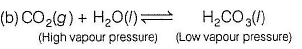When a gas dissolves in H2O, vapour pressure decreases. Hence, increase in pressure of C02 leads to increase in solubility. Hence, soft drink bottles are sealed under high pressure of C02.
Thus, both Statements I are II are correct but Statement II is not the correct explanation of Statement I.

QUESTION: 17

Statement I Scuba (instrum ent used for breathing) divers must cope with high concentration of dissolved gases while breathing at high pressure under water.

Statement II Formation of bubbles in blood blocks capillaries and creates bends which are painful and dangerous to life.

Solution:

(a) When divers breathe under high pressure, solubility of atmospheric gases in blood increases. When divers come at the surface, pressure gradually decreases. Gases (N2 and 02) are released in the form of bubbles which blocks capillaries and creates a medical symptoms called bends which are painful and dangerous. To avoid bends as well as toxic effects of high concentration of nitrogen in the blood, the tanks used by divers are filled with air diluted with helium (11.7% He, 56.2% N2 and 32.1% Og). Thus, both Statements I and II are correct and Statement II is the correct explanation of Statement I.

QUESTION: 18

Matching List Type
Choices for the correct combination of elements from Column I and Column II are given as options (a), (b), (c) and (d), out of which one is correct

The major components of air are 02 and N2 with approximate proportion of 21% and 79% by volume at 298 K. The water is in equilibrium with air at a pressure of 10 atm. At 298 K, KH(N2 ) = 6.5 1 x 107 mm, KH( 02 ) = 3.30 x 107 mm Match the parameters in Column I with their values in Column II and select the answer from the codes given below.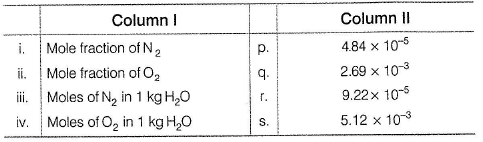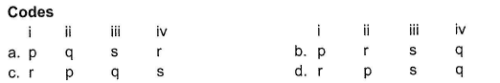Solution: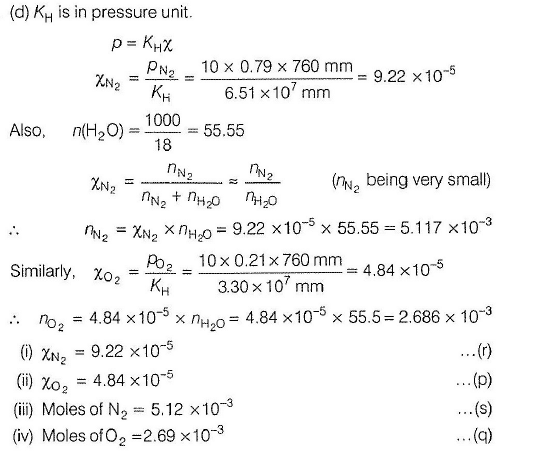QUESTION: 19

Comprehension Type
This section contains a passage describing theory, experiments, data, etc. Two questions related to the paragraph have been given. Each question has only one correct answer out of the given 4 options (a), (b), (c) and (d)

Passage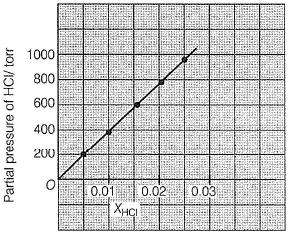Q. Henry’s law constant for HCI gas is (in torr)

Solution:

Based on given figure, pressure of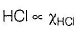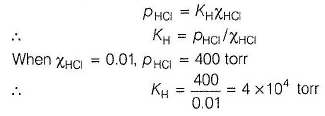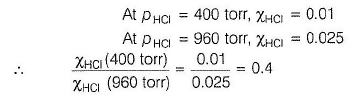QUESTION: 20

The value for Henry’s constant for helium, hydrogen, nitrogen and oxygen at 293 K are 144.97 kbar, 69.16 kbar, 76.48 kbar and 34.86 kbar respectively. Which of the gas will be having maximum solubility?

Solution:

The correct answer is option B.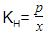At a constant pressure KH is inversely proportional to x therefore higher the KH lower the solubility.
The increasing solubility order is:
He < N2 < H2 < O2

QUESTION: 21

A solution in which no more solute can be dissolved at a given temperature and pressure is called a saturated solution. At saturated solution stage equilibrium gets established among which two processes?

Solution:

At saturated solution stage equilibrium gets established among dissolution and crystallisation.

QUESTION: 22

A solution in which no more solute can be dissolved at the given temperature and pressure is called a

Solution:

The correct answer is option  D
In a saturated solution, more solute cannot be dissolved at a given temperature.
This is because, the solute dissolves in a solvent because of space between particles of solvent but on continuous addition of solute, the space between the solvent particles gets fulfilled. Thus no more solute particle can dissolve in a solvent.

QUESTION: 23

Which expression correctly represents Henry’s Law?

Solution:

The correct answer is option A
Henry’s law is a gas law which states that the amount of gas that is dissolved in a liquid is directly proportional to the partial pressure of that gas above the liquid when the temperature is kept constant. The constant of proportionality for this relationship is called Henry’s law constant (usually denoted by ‘kH‘). The mathematical formula of Henry’s law is given by:
P ∝ C (or) P = kH.C
Where,

• ‘P’ denotes the partial pressure of the gas in the atmosphere above the liquid.

• ‘C’ denotes the concentration of the dissolved gas.

• ‘kH’ is the Henry’s law constant of the gas.

This law was formulated in the early 19th century by the English chemist William Henry. It can be noted that Henry's law constant can be expressed in two different ways. If the constant is defined in terms of solubility/pressure, it is referred to as the Henry’s law solubility constant (denoted by ‘H’). On the other hand, if the proportionality constant is defined in terms of pressure/solubility, it is called the Henry’s law volatility constant (denoted by ‘kH’).

QUESTION: 24

Value of Henry’s constant KH is ______.

Solution:

The correct answer is option A

According to Henry's law, solubility of a gas in a liquid is directly proportional to pressure of gas.
PαX
P = KHX
P = Partial pressure of gas
X = solubility of gas in liquid
KH = Henry's constant
KH depends only on the nature of gas, nature of liquid and temperature (T). As temperature increases, 'KH' increases and 'X' decreases.

QUESTION: 25

Some solute particles in solution collide with other solid solute particles present and get separated out of solution. This process is known as

Solution:
QUESTION: 26

The value for Henry’s constant for argon, carbon dioxide, methane and vinyl chloride at 298 K are 40.3 kbar, 1.67 kbar, 0.413 kbar and 0.611 kbar respectively. Which of the gas will be having least solubility?

Solution:

The correct answer is Option A
The higher the value of KH, the lower is the solubility of the gas in the liquid. Hence the order of increasing solubility of the gases will be Ar <  CO2<CH4< HCHO

QUESTION: 27

Which of the following gases will be least soluble in water?

Solution:
QUESTION: 28

According to Henry’s Law at a constant temperature the solubility of gas in a liquid is directly proportional to the

Solution:

The correct answer is option D
Henry's law states that at a constant temperature, the solubility of a gas is directly proportional to the pressure of the gas.
In other words, The partial pressure of the gas in vapour phase (p) is proportional to the mole fraction of the gas (x) in the solution.
p=KH​ x
Here, KH​ is Henry's law constant.
Hence, the given statement is Henry's law.

QUESTION: 29

When a large amount of solute dissolves in a given amount of solvent it forms

Solution: